Скачать презентацию Ionosphere and Neutral Atmosphere Temperature and density

5263ca90fc64d4572bc9d6d9391c9830.ppt

• Количество слайдов: 58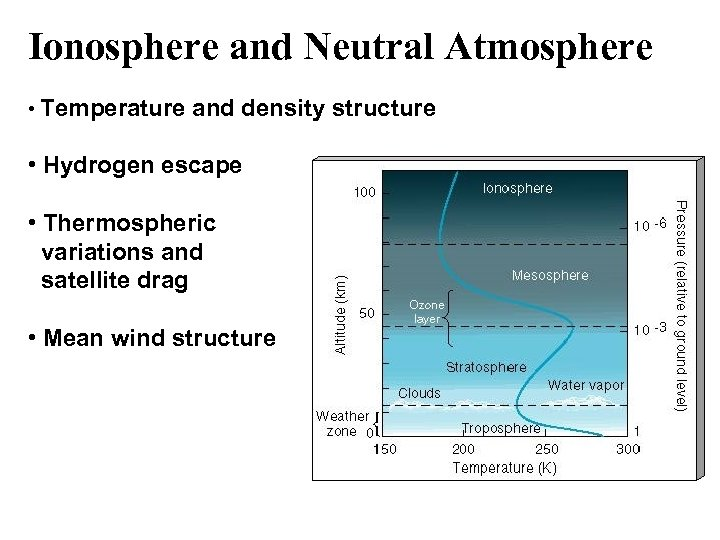Ionosphere and Neutral Atmosphere • Temperature and density structure • Hydrogen escape • Thermospheric variations and satellite drag • Mean wind structure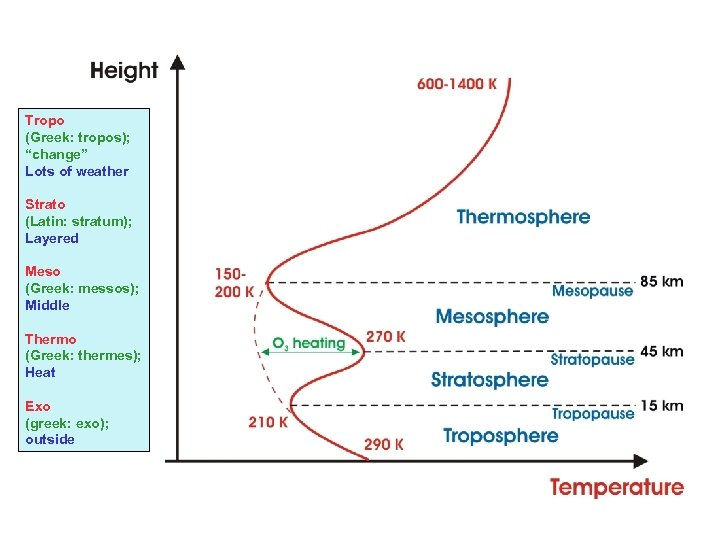Tropo (Greek: tropos); “change” Lots of weather Strato (Latin: stratum); Layered Meso (Greek: messos); Middle Thermo (Greek: thermes); Heat Exo (greek: exo); outside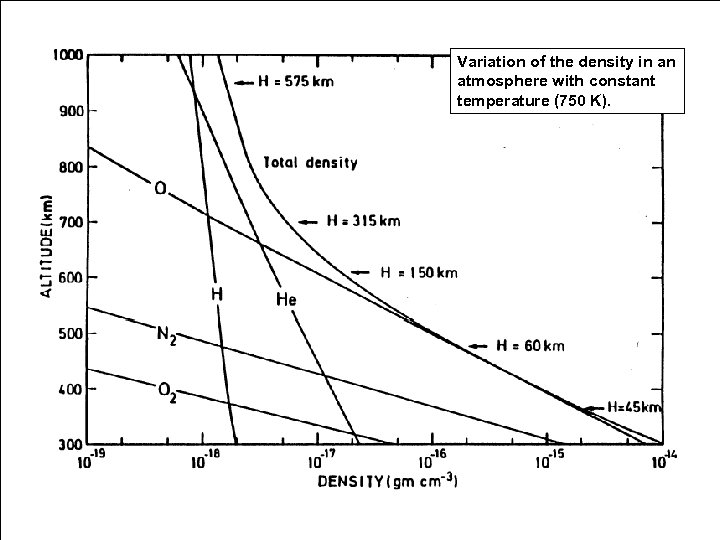Variation of the density in an atmosphere with constant temperature (750 K).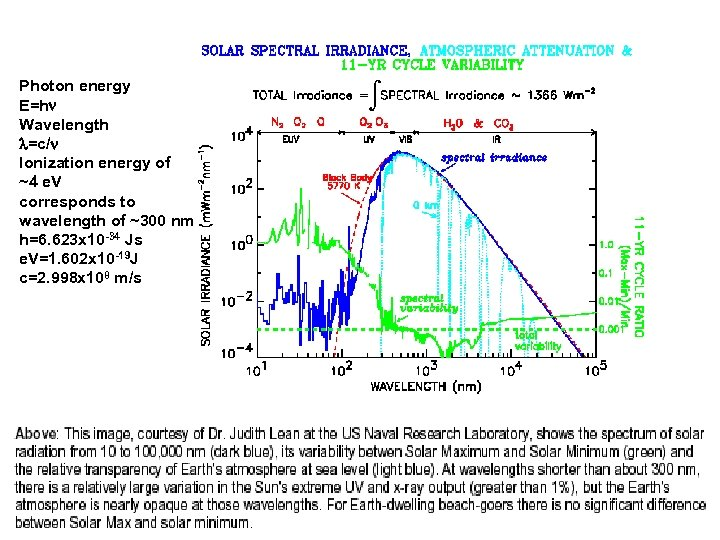Photon energy E=h Wavelength =c/ Ionization energy of ~4 e. V corresponds to wavelength of ~300 nm h=6. 623 x 10 -34 Js e. V=1. 602 x 10 -19 J c=2. 998 x 108 m/s 4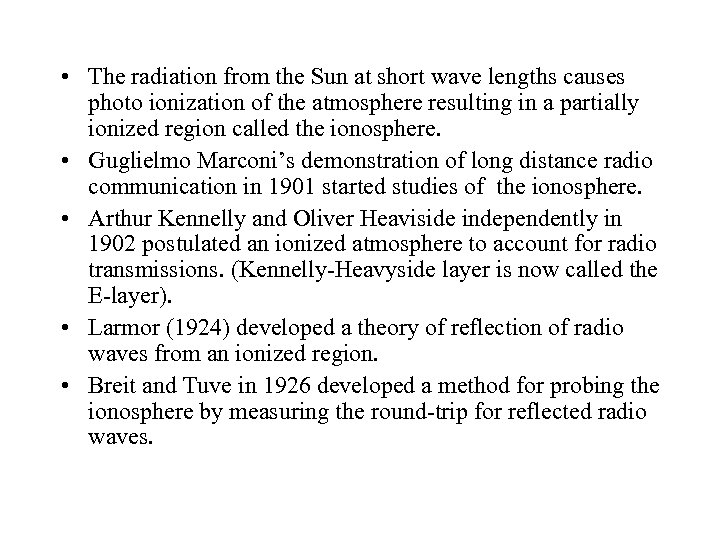• The radiation from the Sun at short wave lengths causes photo ionization of the atmosphere resulting in a partially ionized region called the ionosphere. • Guglielmo Marconi’s demonstration of long distance radio communication in 1901 started studies of the ionosphere. • Arthur Kennelly and Oliver Heaviside independently in 1902 postulated an ionized atmosphere to account for radio transmissions. (Kennelly-Heavyside layer is now called the E-layer). • Larmor (1924) developed a theory of reflection of radio waves from an ionized region. • Breit and Tuve in 1926 developed a method for probing the ionosphere by measuring the round-trip for reflected radio waves.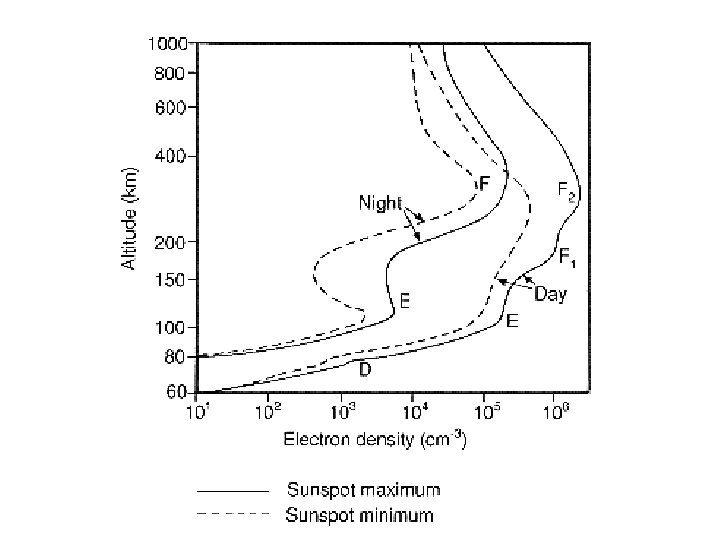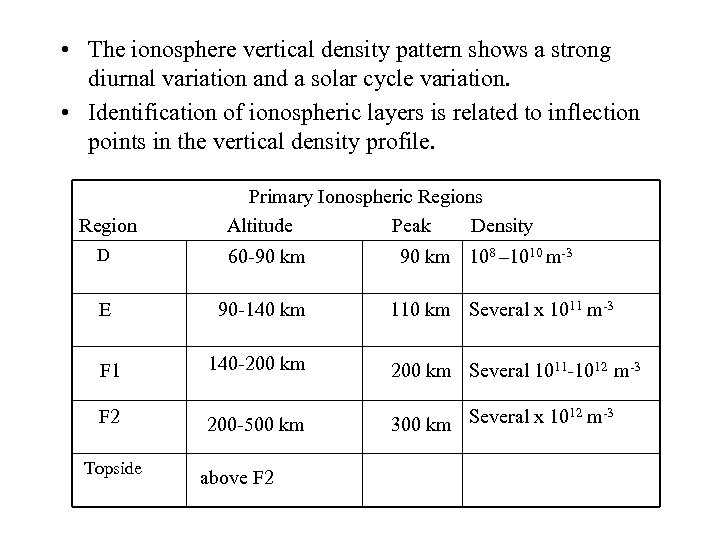• The ionosphere vertical density pattern shows a strong diurnal variation and a solar cycle variation. • Identification of ionospheric layers is related to inflection points in the vertical density profile. Region Primary Ionospheric Regions Altitude Peak Density D 60 -90 km E 90 -140 km F 1 140 -200 km F 2 200 -500 km Topside above F 2 90 km 108 – 1010 m-3 110 km Several x 1011 m-3 200 km Several 1011 -1012 m-3 12 -3 300 km Several x 10 m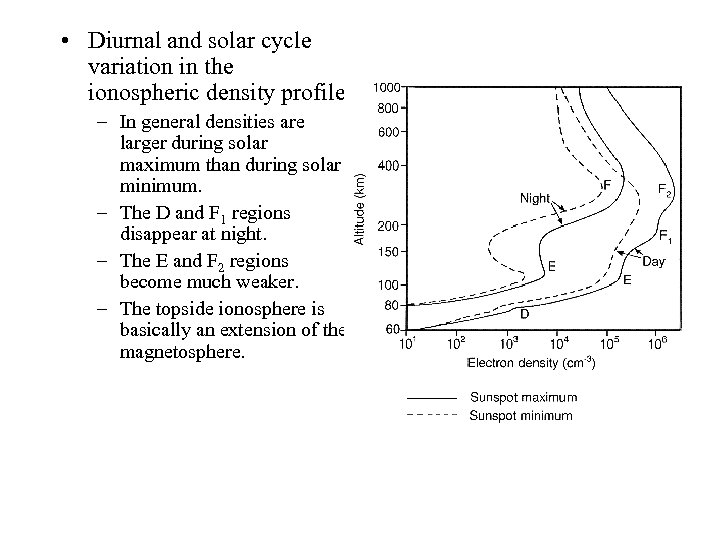• Diurnal and solar cycle variation in the ionospheric density profile. – In general densities are larger during solar maximum than during solar minimum. – The D and F 1 regions disappear at night. – The E and F 2 regions become much weaker. – The topside ionosphere is basically an extension of the magnetosphere.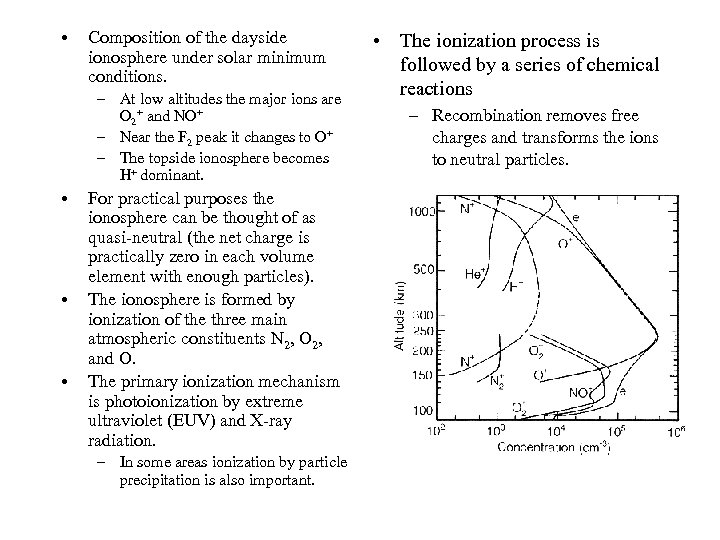• Composition of the dayside ionosphere under solar minimum conditions. – At low altitudes the major ions are O 2+ and NO+ – Near the F 2 peak it changes to O+ – The topside ionosphere becomes H+ dominant. • • • For practical purposes the ionosphere can be thought of as quasi-neutral (the net charge is practically zero in each volume element with enough particles). The ionosphere is formed by ionization of the three main atmospheric constituents N 2, O 2, and O. The primary ionization mechanism is photoionization by extreme ultraviolet (EUV) and X-ray radiation. – In some areas ionization by particle precipitation is also important. • The ionization process is followed by a series of chemical reactions – Recombination removes free charges and transforms the ions to neutral particles.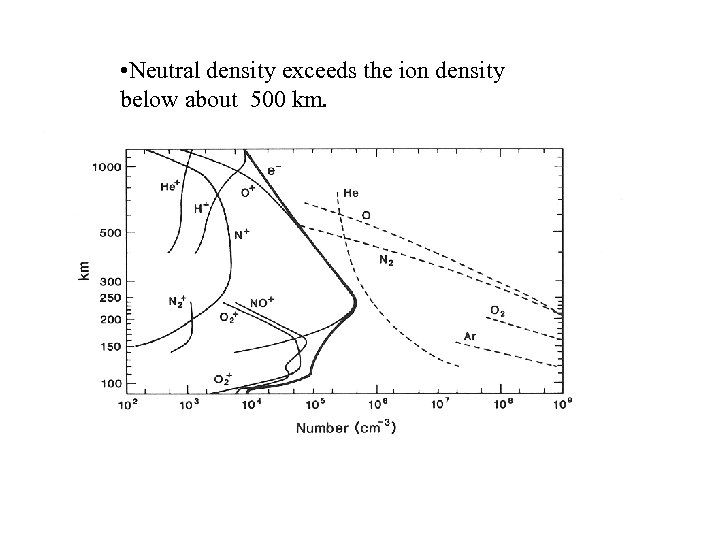• Neutral density exceeds the ion density below about 500 km.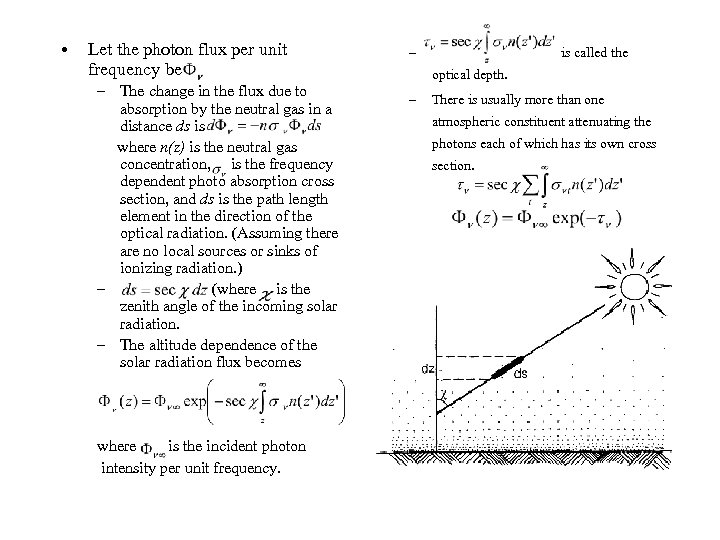• Let the photon flux per unit frequency be – The change in the flux due to absorption by the neutral gas in a distance ds is where n(z) is the neutral gas concentration, is the frequency dependent photo absorption cross section, and ds is the path length element in the direction of the optical radiation. (Assuming there are no local sources or sinks of ionizing radiation. ) – (where is the zenith angle of the incoming solar radiation. – The altitude dependence of the solar radiation flux becomes where is the incident photon intensity per unit frequency. – is called the optical depth. – There is usually more than one atmospheric constituent attenuating the photons each of which has its own cross section.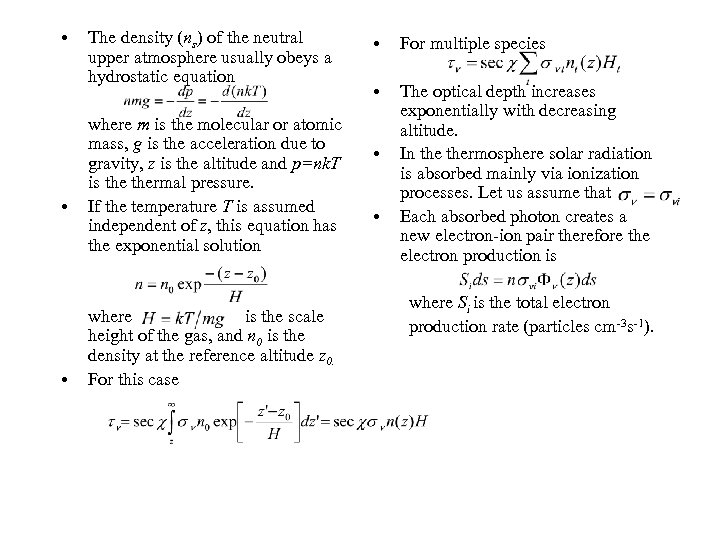• • • The density (ns) of the neutral upper atmosphere usually obeys a hydrostatic equation where m is the molecular or atomic mass, g is the acceleration due to gravity, z is the altitude and p=nk. T is thermal pressure. If the temperature T is assumed independent of z, this equation has the exponential solution where is the scale height of the gas, and n 0 is the density at the reference altitude z 0. For this case • For multiple species • The optical depth increases exponentially with decreasing altitude. In thermosphere solar radiation is absorbed mainly via ionization processes. Let us assume that Each absorbed photon creates a new electron-ion pair therefore the electron production is • • where Si is the total electron production rate (particles cm-3 s-1).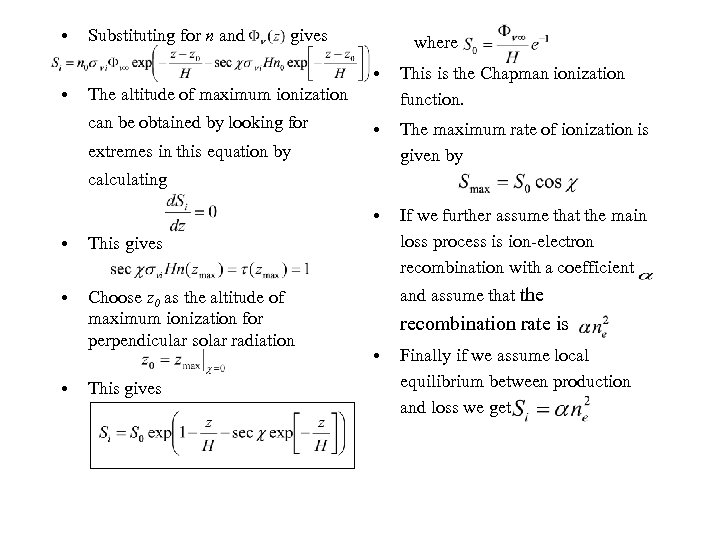• Substituting for n and gives where • • The maximum rate of ionization is given by • • This is the Chapman ionization function. If we further assume that the main loss process is ion-electron recombination with a coefficient The altitude of maximum ionization can be obtained by looking for extremes in this equation by calculating • This gives • Choose z 0 as the altitude of maximum ionization for perpendicular solar radiation • This gives and assume that the recombination rate is • Finally if we assume local equilibrium between production and loss we get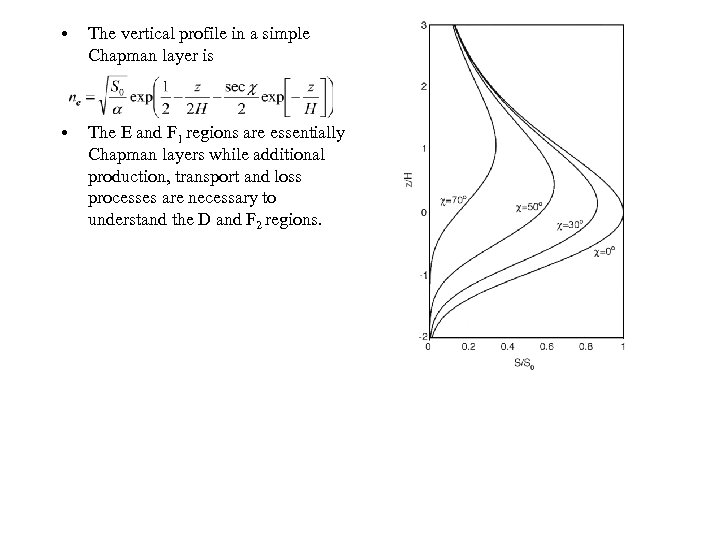• The vertical profile in a simple Chapman layer is • The E and F 1 regions are essentially Chapman layers while additional production, transport and loss processes are necessary to understand the D and F 2 regions.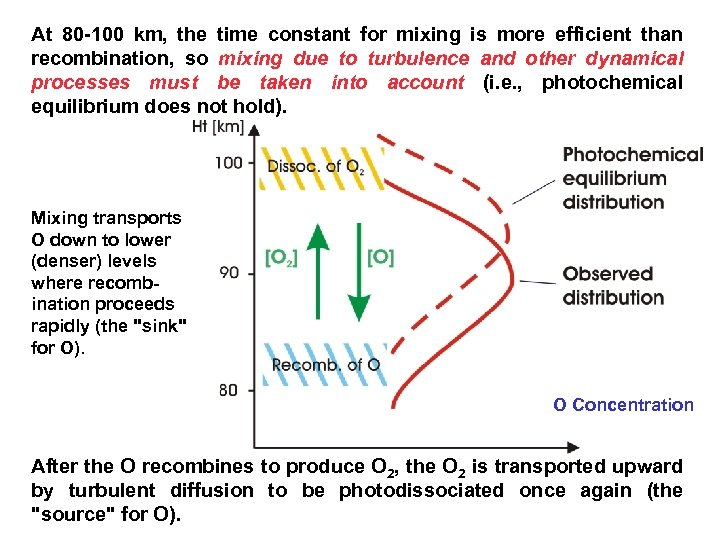At 80 -100 km, the time constant for mixing is more efficient than recombination, so mixing due to turbulence and other dynamical processes must be taken into account (i. e. , photochemical equilibrium does not hold). Mixing transports O down to lower (denser) levels where recombination proceeds rapidly (the "sink" for O). O Concentration After the O recombines to produce O 2, the O 2 is transported upward by turbulent diffusion to be photodissociated once again (the "source" for O).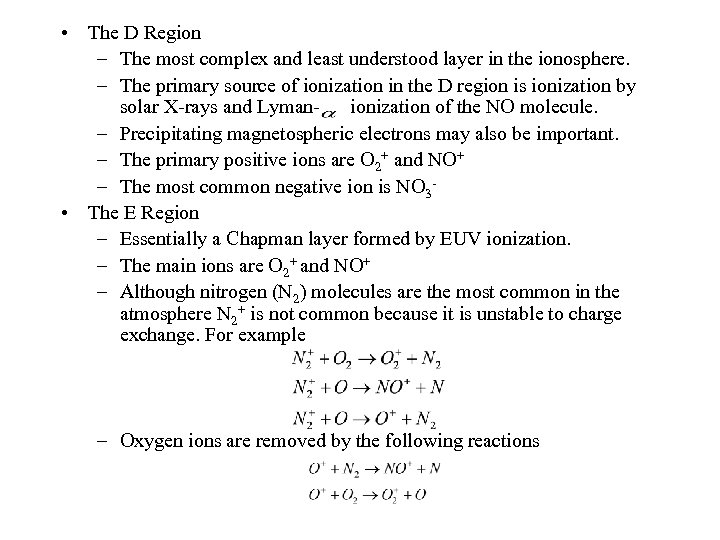• The D Region – The most complex and least understood layer in the ionosphere. – The primary source of ionization in the D region is ionization by solar X-rays and Lymanionization of the NO molecule. – Precipitating magnetospheric electrons may also be important. – The primary positive ions are O 2+ and NO+ – The most common negative ion is NO 3 • The E Region – Essentially a Chapman layer formed by EUV ionization. – The main ions are O 2+ and NO+ – Although nitrogen (N 2) molecules are the most common in the atmosphere N 2+ is not common because it is unstable to charge exchange. For example – Oxygen ions are removed by the following reactions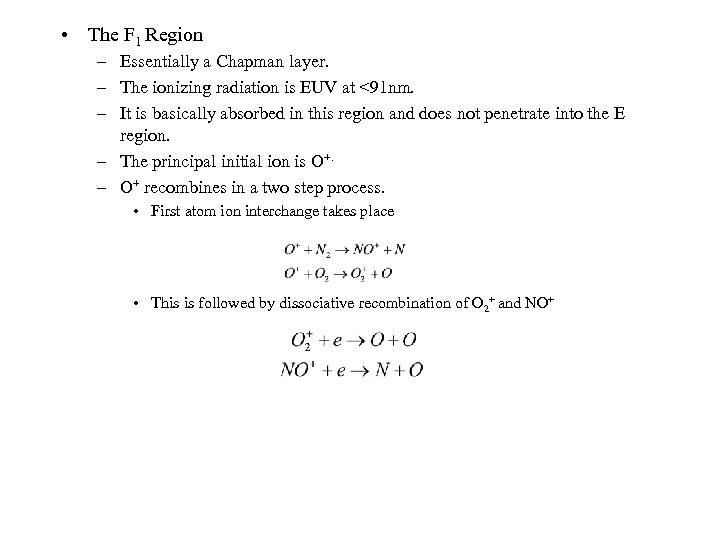• The F 1 Region – Essentially a Chapman layer. – The ionizing radiation is EUV at <91 nm. – It is basically absorbed in this region and does not penetrate into the E region. – The principal initial ion is O+. – O+ recombines in a two step process. • First atom ion interchange takes place • This is followed by dissociative recombination of O 2+ and NO+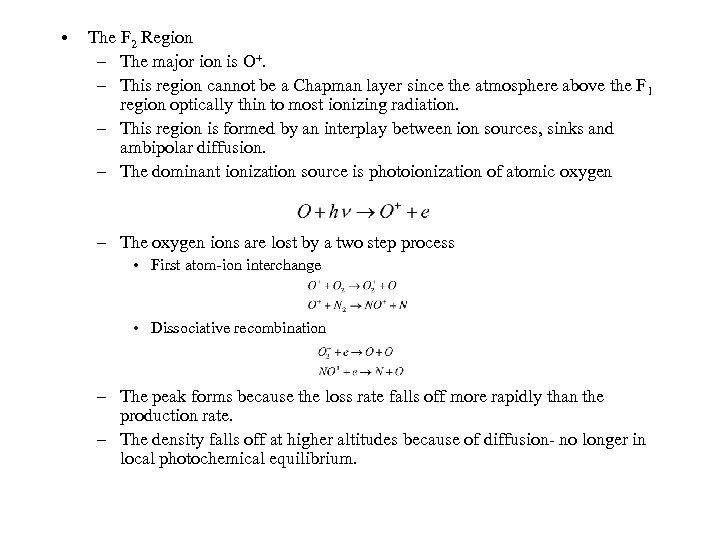• The F 2 Region – The major ion is O+. – This region cannot be a Chapman layer since the atmosphere above the F 1 region optically thin to most ionizing radiation. – This region is formed by an interplay between ion sources, sinks and ambipolar diffusion. – The dominant ionization source is photoionization of atomic oxygen – The oxygen ions are lost by a two step process • First atom-ion interchange • Dissociative recombination – The peak forms because the loss rate falls off more rapidly than the production rate. – The density falls off at higher altitudes because of diffusion- no longer in local photochemical equilibrium.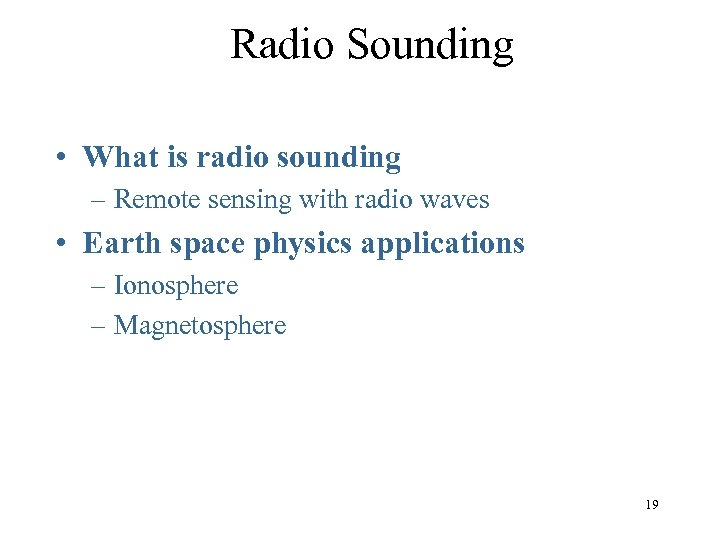Radio Sounding • What is radio sounding – Remote sensing with radio waves • Earth space physics applications – Ionosphere – Magnetosphere 19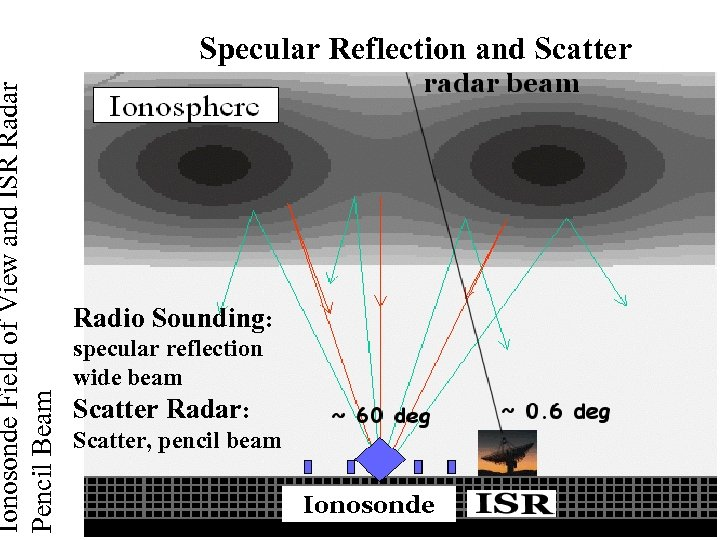Ionosonde Field of View and ISR Radar Pencil Beam Specular Reflection and Scatter Radio Sounding: specular reflection wide beam Scatter Radar: Scatter, pencil beam Ionosonde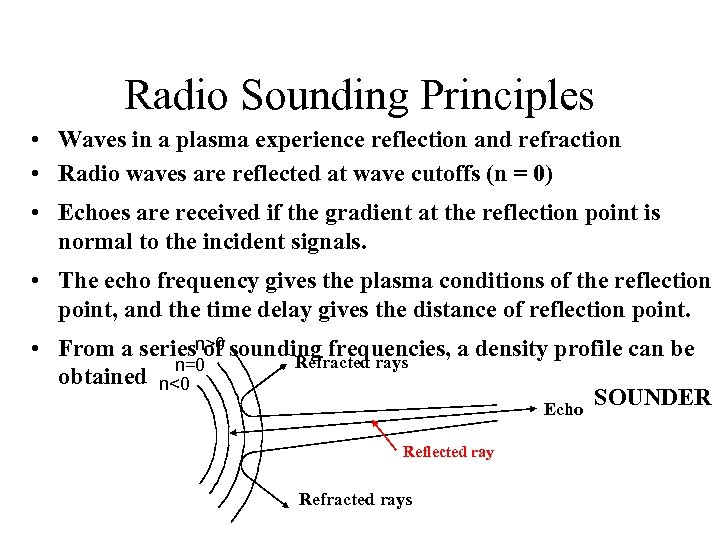Radio Sounding Principles • Waves in a plasma experience reflection and refraction • Radio waves are reflected at wave cutoffs (n = 0) • Echoes are received if the gradient at the reflection point is normal to the incident signals. • The echo frequency gives the plasma conditions of the reflection point, and the time delay gives the distance of reflection point. • From a seriesn>0 sounding frequencies, a density profile can be of Refracted rays n=0 obtained n<0 SOUNDER Echo Reflected ray Refracted rays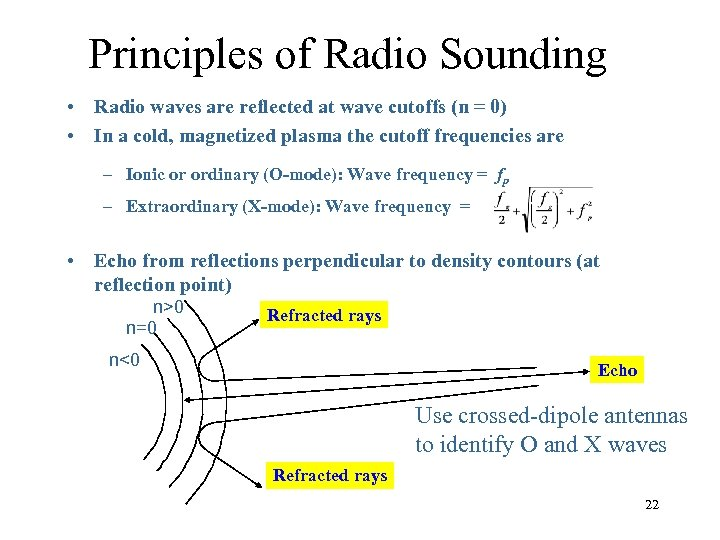Principles of Radio Sounding • Radio waves are reflected at wave cutoffs (n = 0) • In a cold, magnetized plasma the cutoff frequencies are – Ionic or ordinary (O-mode): Wave frequency = fp – Extraordinary (X-mode): Wave frequency = • Echo from reflections perpendicular to density contours (at reflection point) n>0 n=0 Refracted rays n<0 Echo Use crossed-dipole antennas to identify O and X waves Refracted rays 22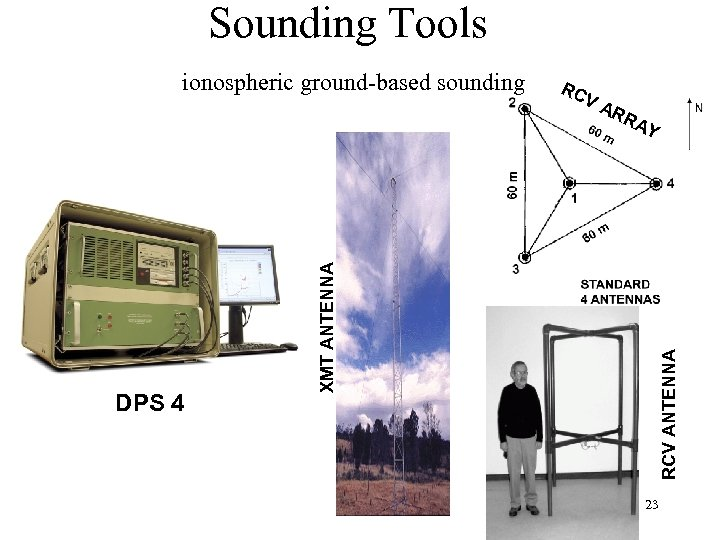Sounding Tools VA RR AY RCV ANTENNA DPS 4 RC XMT ANTENNA ionospheric ground-based sounding 23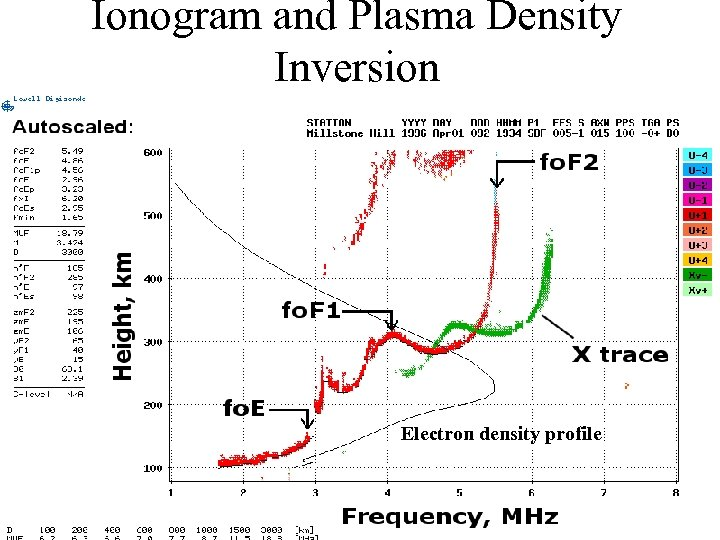Ionogram and Plasma Density Inversion Electron density profile 24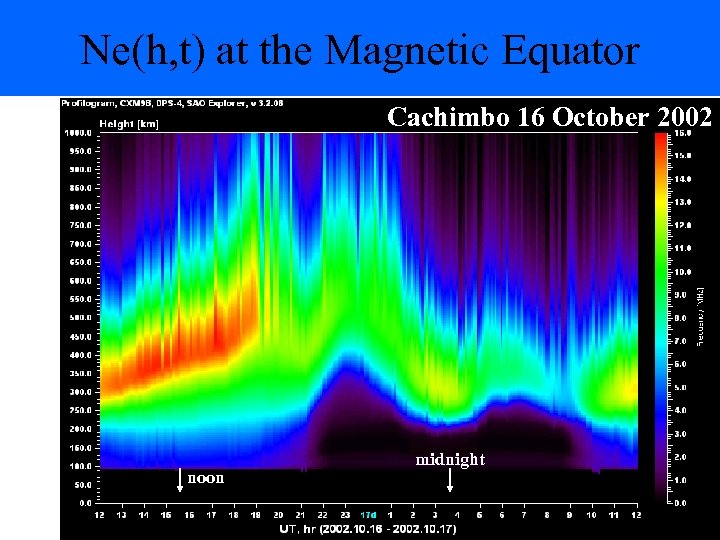Ne(h, t) at the Magnetic Equator Cachimbo 16 October 2002 noon midnight 25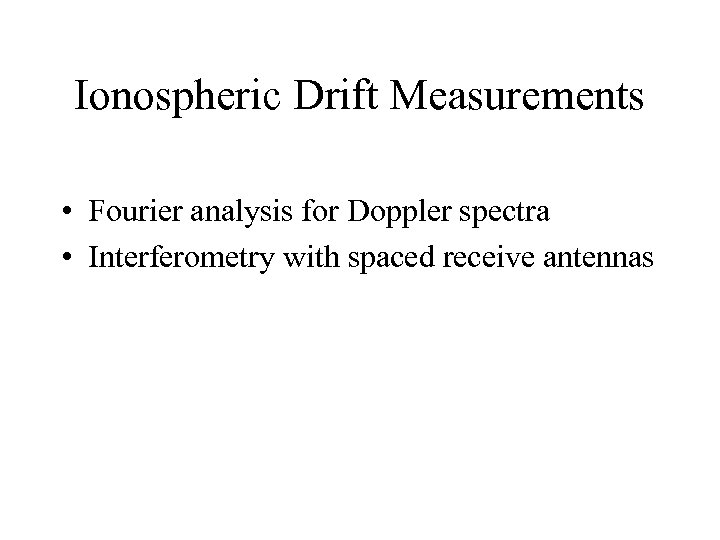Ionospheric Drift Measurements • Fourier analysis for Doppler spectra • Interferometry with spaced receive antennas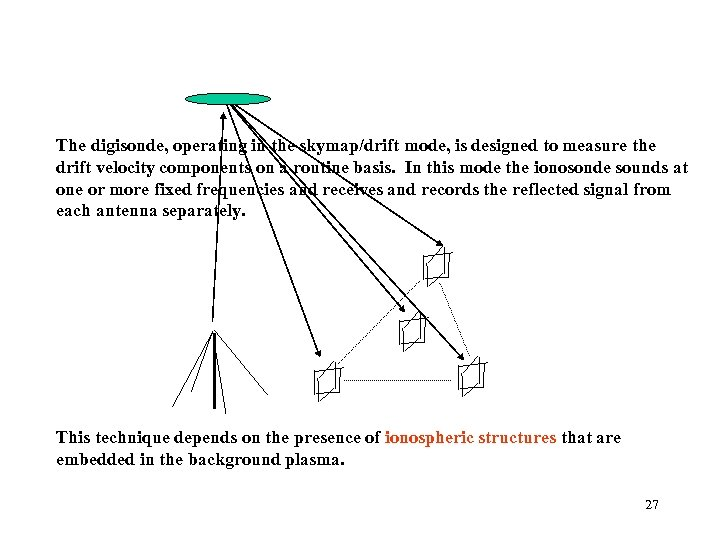Interferometric Doppler Imaging The digisonde, operating in the skymap/drift mode, is designed to measure the drift velocity components on a routine basis. In this mode the ionosonde sounds at one or more fixed frequencies and receives and records the reflected signal from each antenna separately. This technique depends on the presence of ionospheric structures that are embedded in the background plasma. 27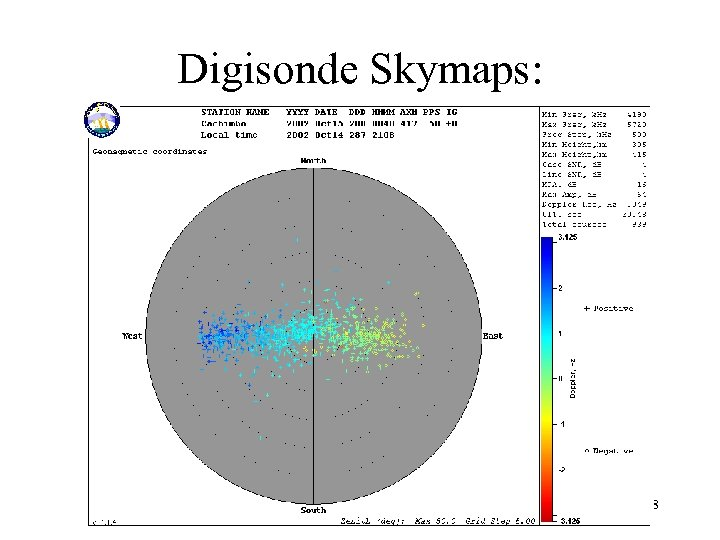Digisonde Skymaps: Reflections and Doppler Shifts 28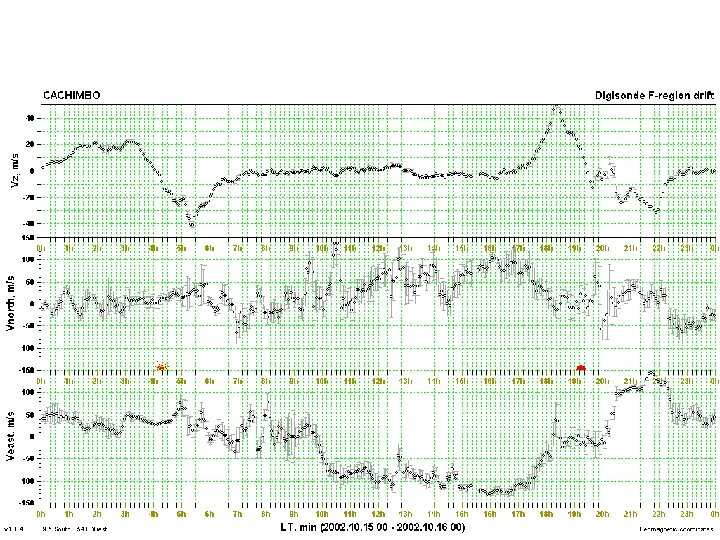Real Time Digisonde F-Region Drift Measurements 29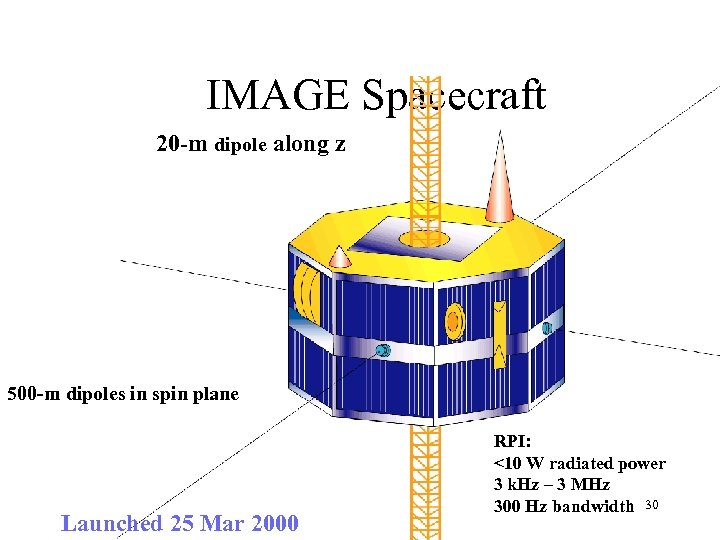IMAGE Spacecraft 20 -m dipole along z 500 -m dipoles in spin plane Launched 25 Mar 2000 RPI: <10 W radiated power 3 k. Hz – 3 MHz 300 Hz bandwidth 30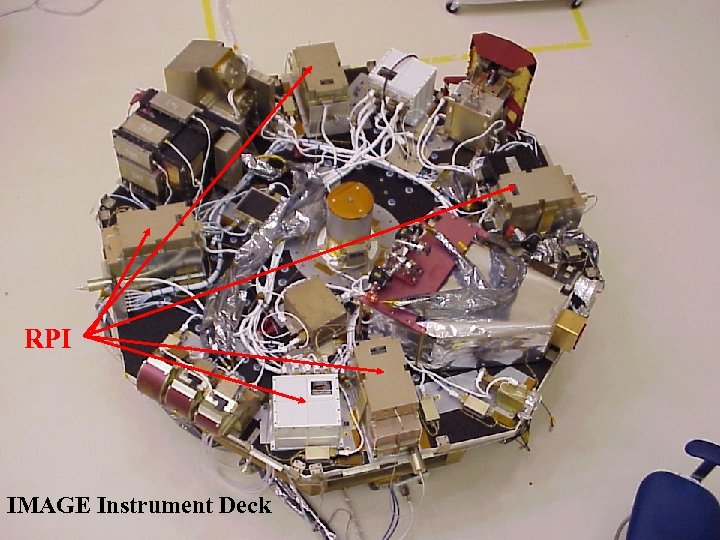RPI IMAGE Instrument Deck 31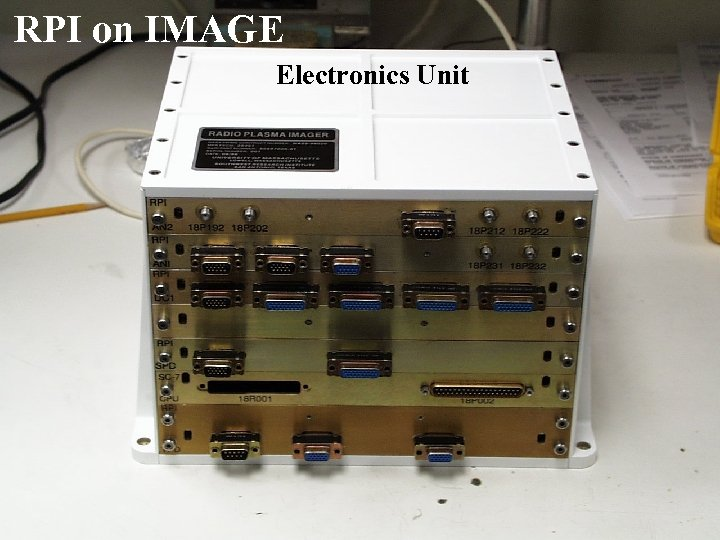RPI on IMAGE Electronics Unit 32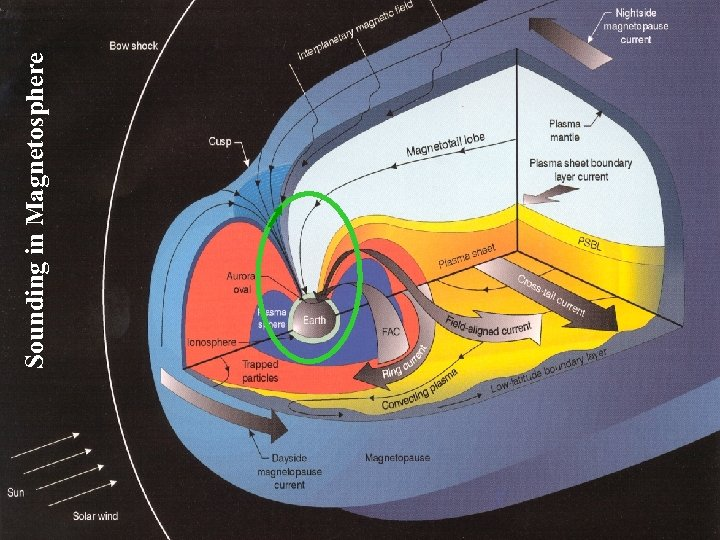33 Sounding in Magnetosphere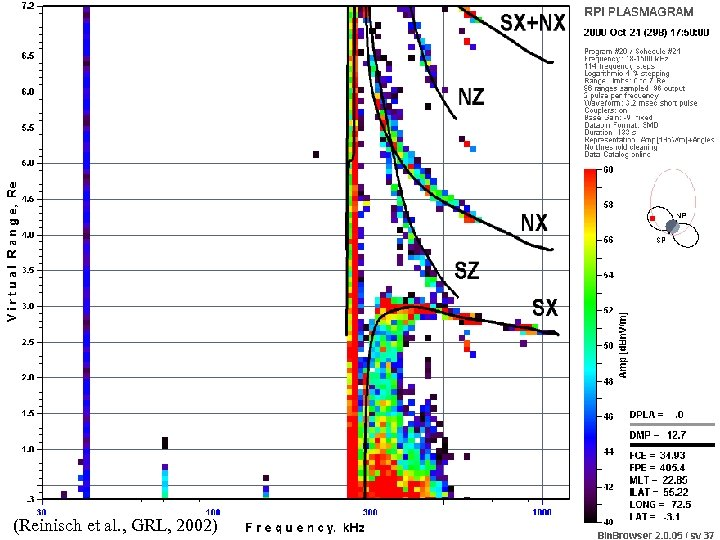Field-Aligned Propagation RPI Plasmagram Fig 2 of GRL paper 34 (Reinisch et al. , GRL, 2002)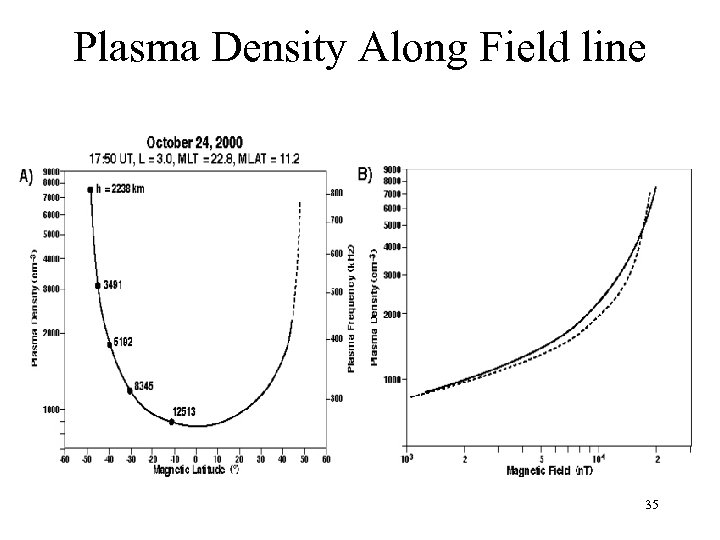Plasma Density Along Field line 35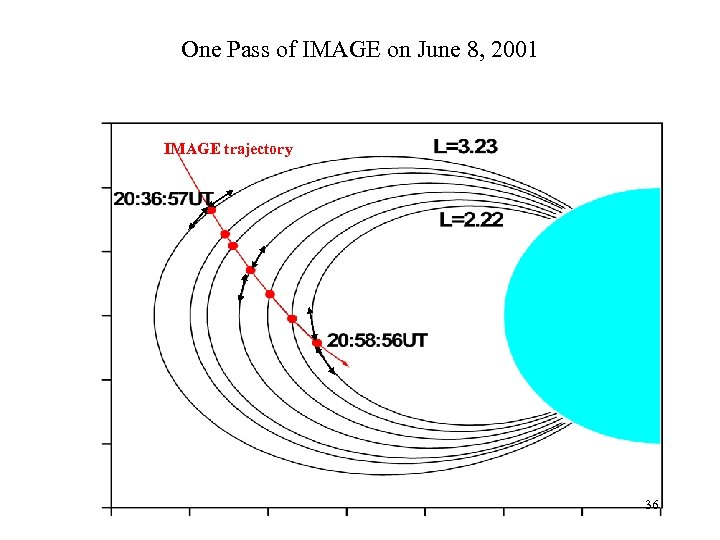One Pass of IMAGE on June 8, 2001 IMAGE trajectory 36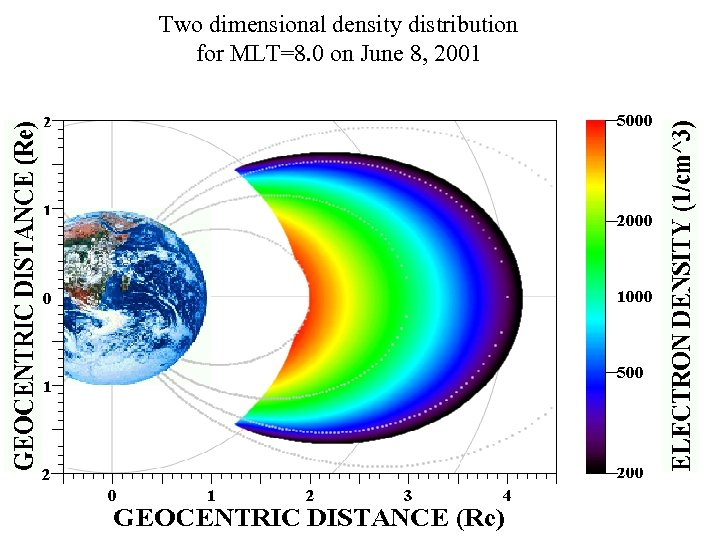Two dimensional density distribution for MLT=8. 0 on June 8, 2001 37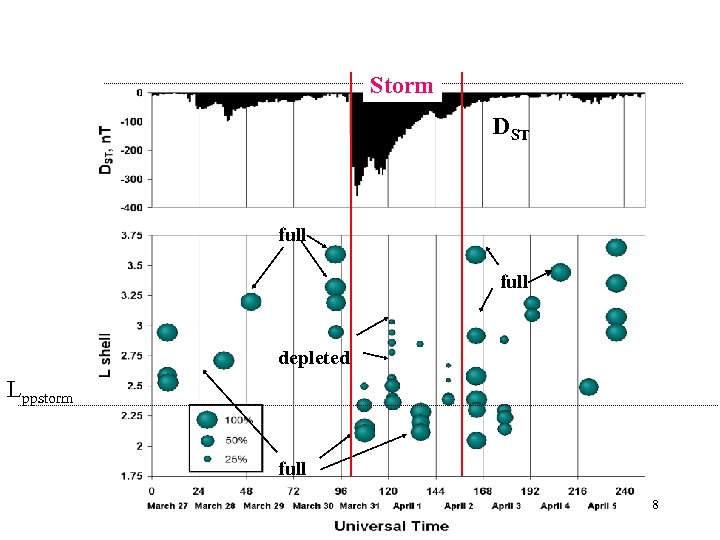Plasmasphere Depleting and Refilling Storm DST full depleted Lppstorm full 38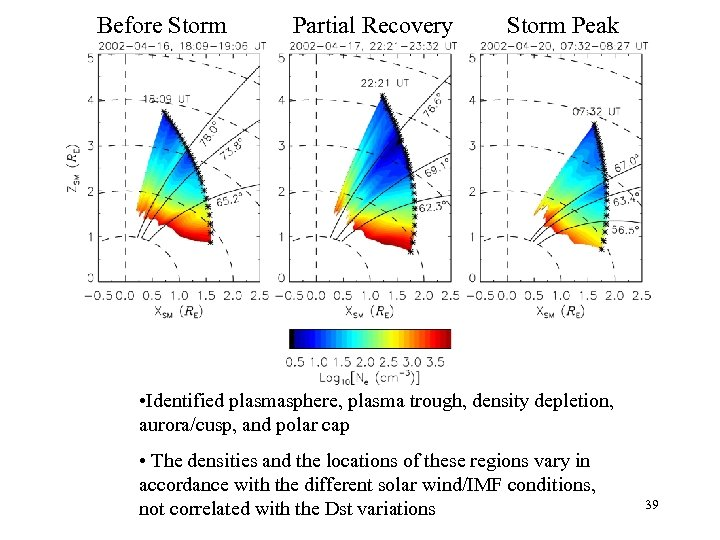Before Storm Partial Recovery Storm Peak • Identified plasmasphere, plasma trough, density depletion, aurora/cusp, and polar cap • The densities and the locations of these regions vary in accordance with the different solar wind/IMF conditions, not correlated with the Dst variations 39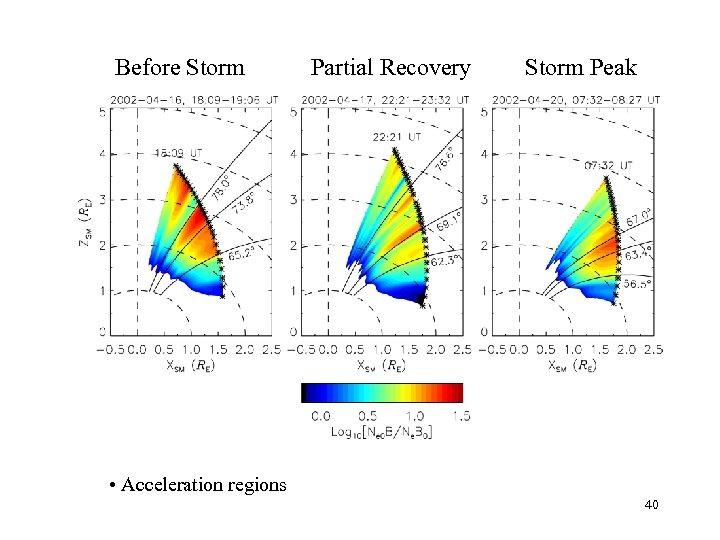Before Storm Partial Recovery Storm Peak • Acceleration regions 40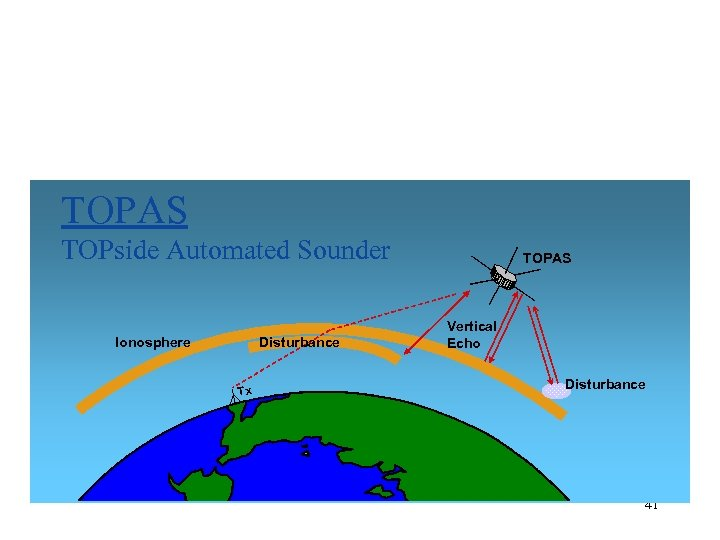Ionospheric Topside Sounding TOPAS TOPside Automated Sounder Ionosphere Disturbance Tx TOPAS Vertical Echo Disturbance 41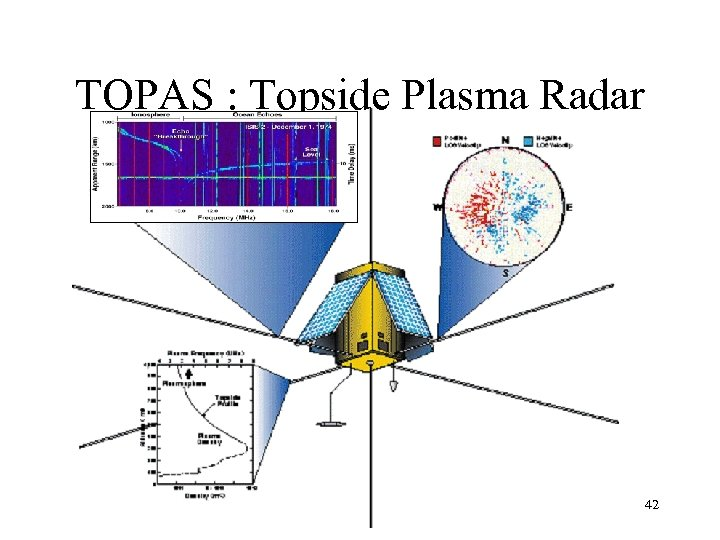TOPAS : Topside Plasma Radar 42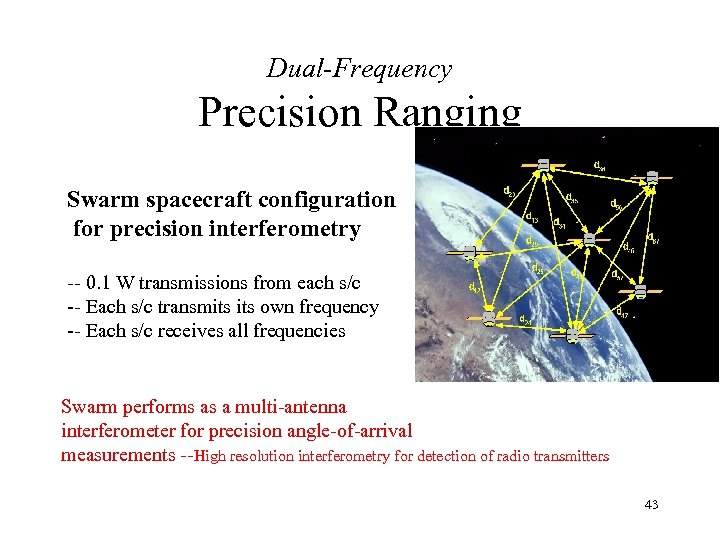Dual-Frequency Precision Ranging Swarm spacecraft configuration for precision interferometry -- 0. 1 W transmissions from each s/c -- Each s/c transmits own frequency -- Each s/c receives all frequencies Swarm performs as a multi-antenna interferometer for precision angle-of-arrival measurements --High resolution interferometry for detection of radio transmitters 43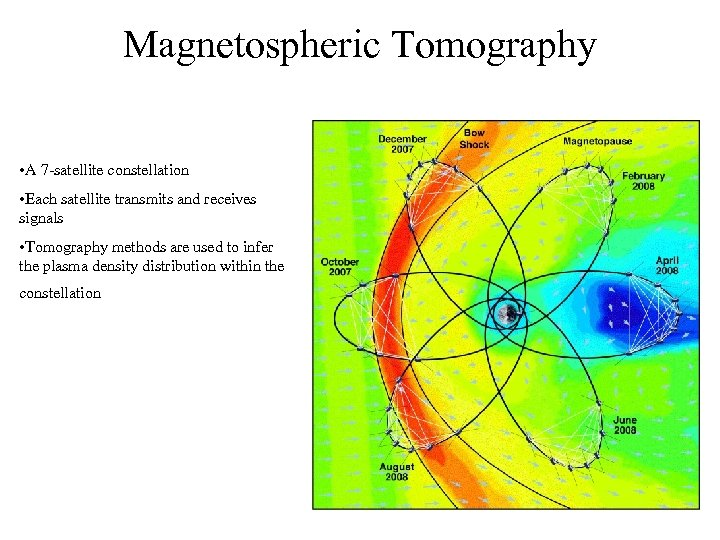Magnetospheric Tomography • A 7 -satellite constellation • Each satellite transmits and receives signals • Tomography methods are used to infer the plasma density distribution within the constellation 44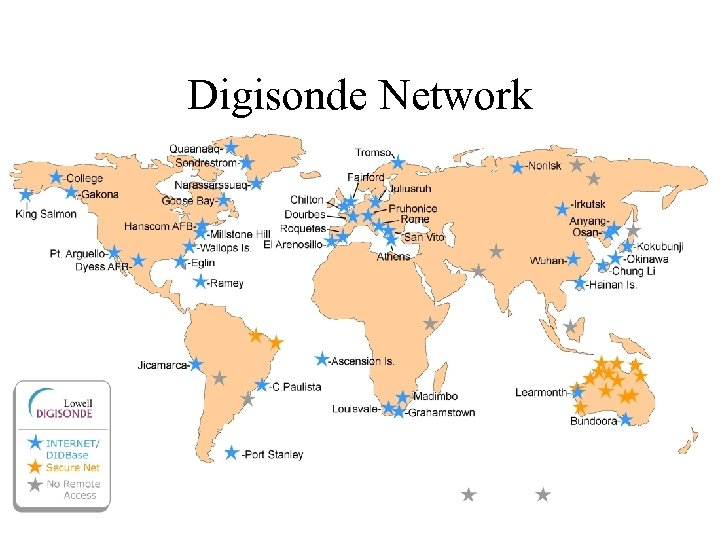Digisonde Network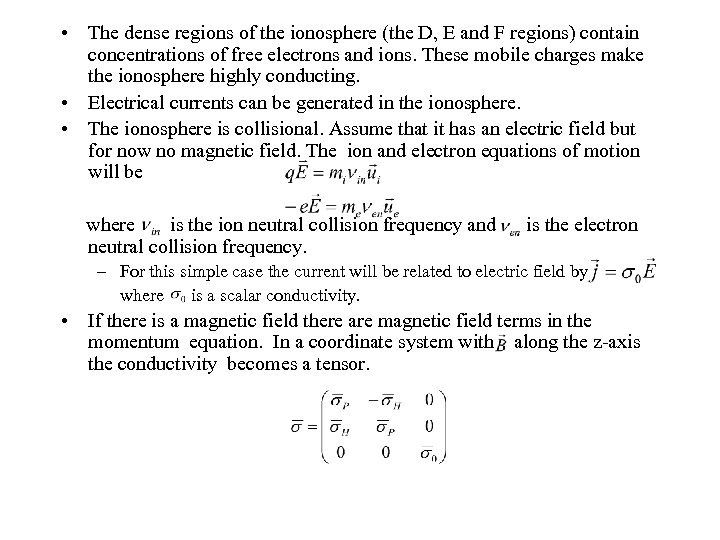• The dense regions of the ionosphere (the D, E and F regions) contain concentrations of free electrons and ions. These mobile charges make the ionosphere highly conducting. • Electrical currents can be generated in the ionosphere. • The ionosphere is collisional. Assume that it has an electric field but for now no magnetic field. The ion and electron equations of motion will be where is the ion neutral collision frequency and neutral collision frequency. is the electron – For this simple case the current will be related to electric field by where is a scalar conductivity. • If there is a magnetic field there are magnetic field terms in the momentum equation. In a coordinate system with along the z-axis the conductivity becomes a tensor.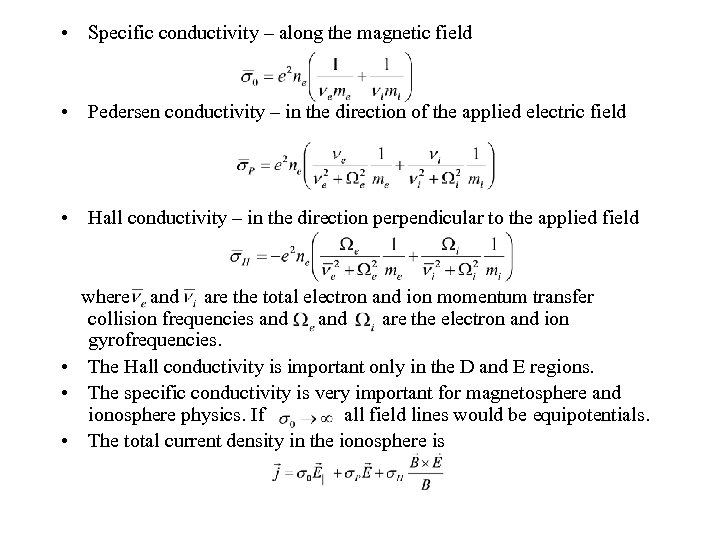• Specific conductivity – along the magnetic field • Pedersen conductivity – in the direction of the applied electric field • Hall conductivity – in the direction perpendicular to the applied field where and are the total electron and ion momentum transfer collision frequencies and are the electron and ion gyrofrequencies. • The Hall conductivity is important only in the D and E regions. • The specific conductivity is very important for magnetosphere and ionosphere physics. If all field lines would be equipotentials. • The total current density in the ionosphere is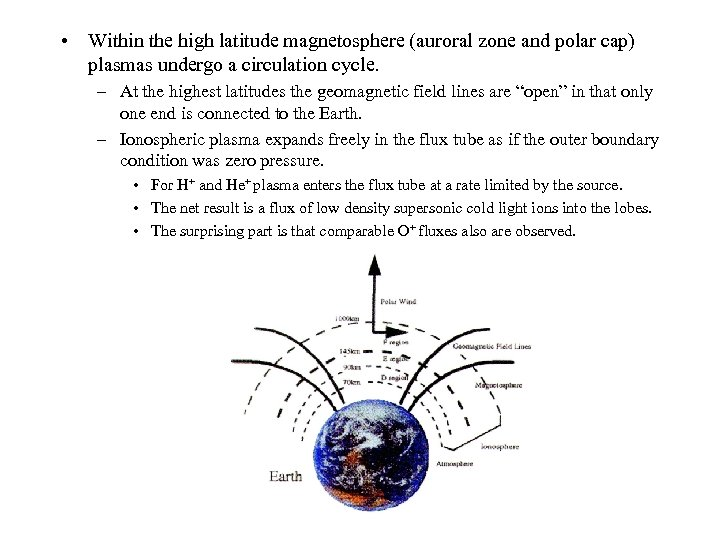• Within the high latitude magnetosphere (auroral zone and polar cap) plasmas undergo a circulation cycle. – At the highest latitudes the geomagnetic field lines are “open” in that only one end is connected to the Earth. – Ionospheric plasma expands freely in the flux tube as if the outer boundary condition was zero pressure. • For H+ and He+ plasma enters the flux tube at a rate limited by the source. • The net result is a flux of low density supersonic cold light ions into the lobes. • The surprising part is that comparable O+ fluxes also are observed.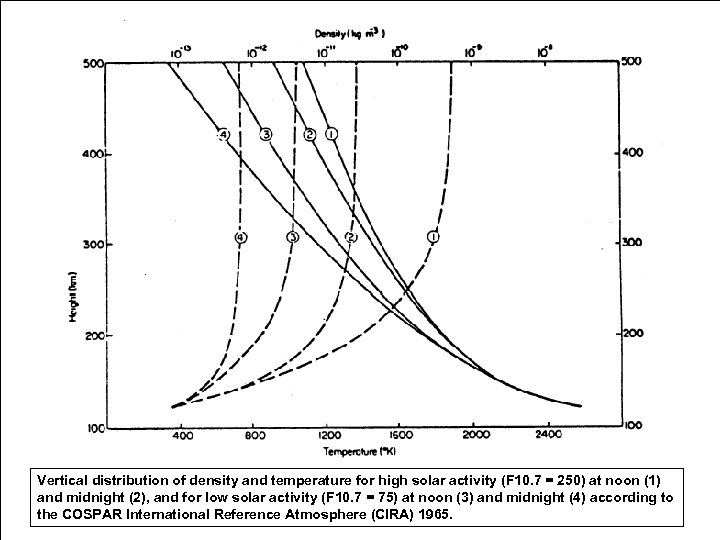Vertical distribution of density and temperature for high solar activity (F 10. 7 = 250) at noon (1) and midnight (2), and for low solar activity (F 10. 7 = 75) at noon (3) and midnight (4) according to the COSPAR International Reference Atmosphere (CIRA) 1965.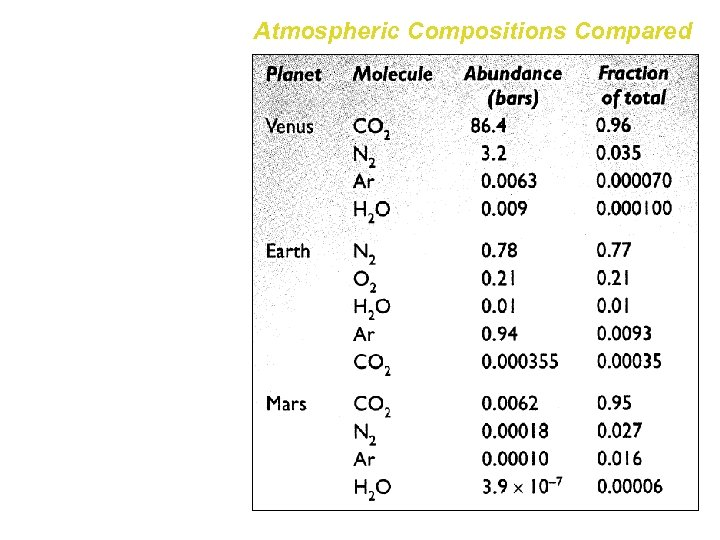Atmospheric Compositions Compared The atmospheres of Earth, Venus and Mars contain many of the same gases, but in very different absolute and relative abundances. Some values are lower limits only, reflecting the past escape of gas to space and other factors.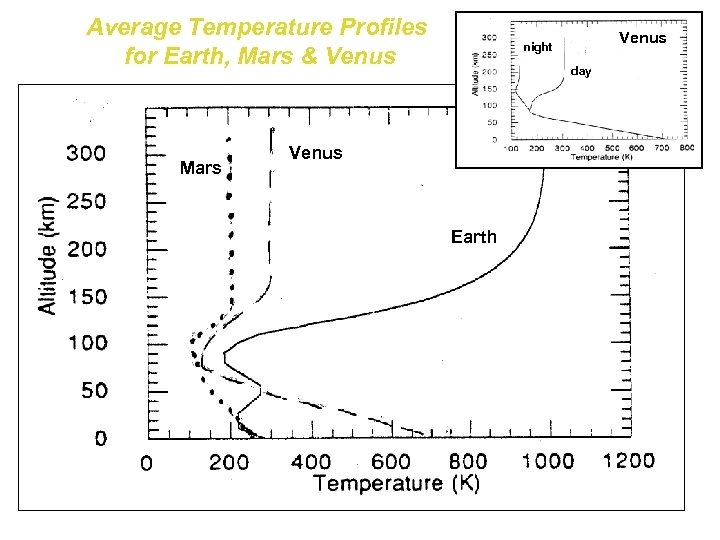Average Temperature Profiles for Earth, Mars & Venus Mars Venus night day Venus Earth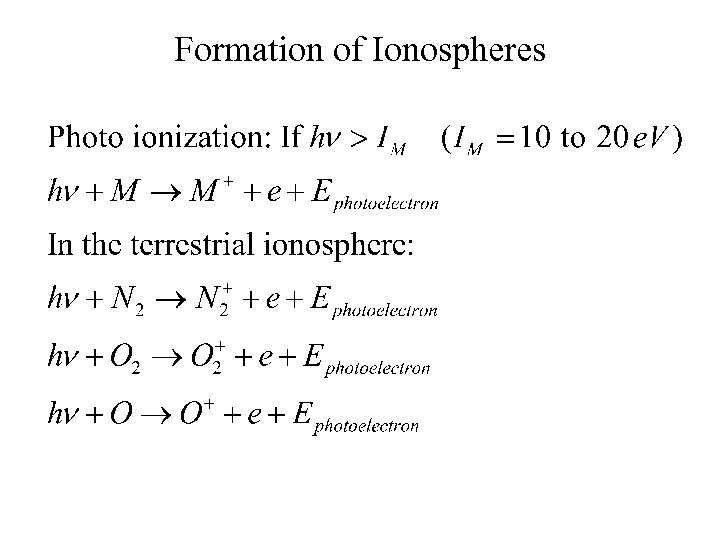Formation of Ionospheres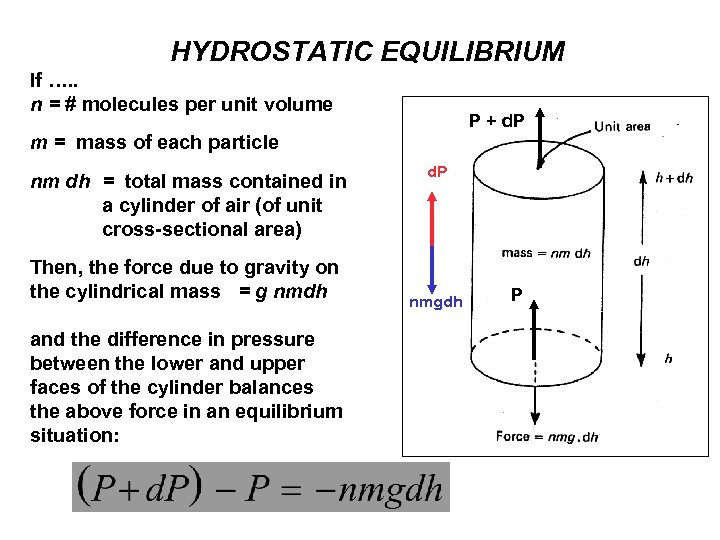HYDROSTATIC EQUILIBRIUM If …. . n = # molecules per unit volume P + d. P m = mass of each particle nm dh = total mass contained in a cylinder of air (of unit cross-sectional area) Then, the force due to gravity on the cylindrical mass = g nmdh and the difference in pressure between the lower and upper faces of the cylinder balances the above force in an equilibrium situation: d. P nmgdh P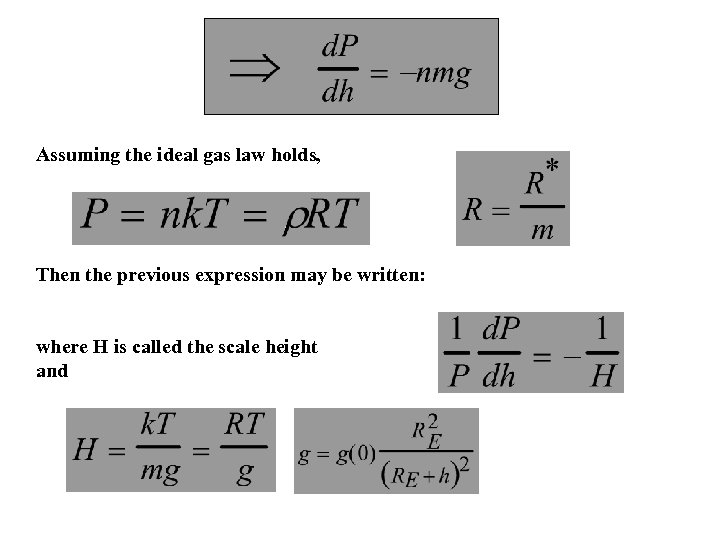Assuming the ideal gas law holds, Then the previous expression may be written: where H is called the scale height and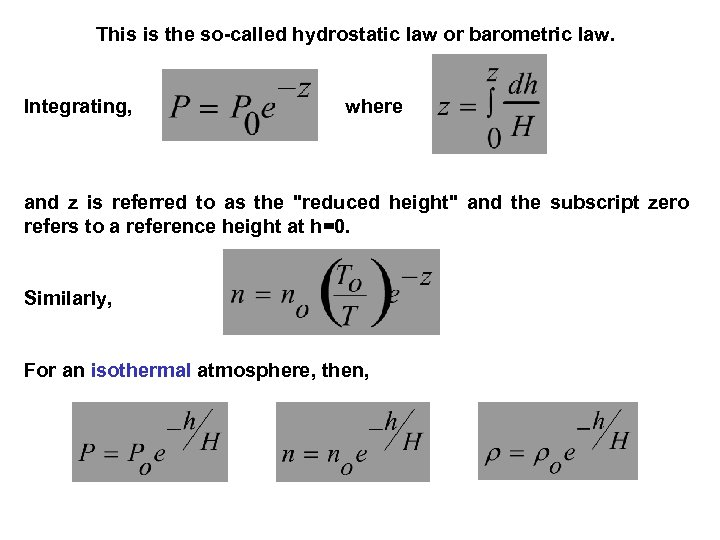This is the so-called hydrostatic law or barometric law. Integrating, where and z is referred to as the "reduced height" and the subscript zero refers to a reference height at h=0. Similarly, For an isothermal atmosphere, then,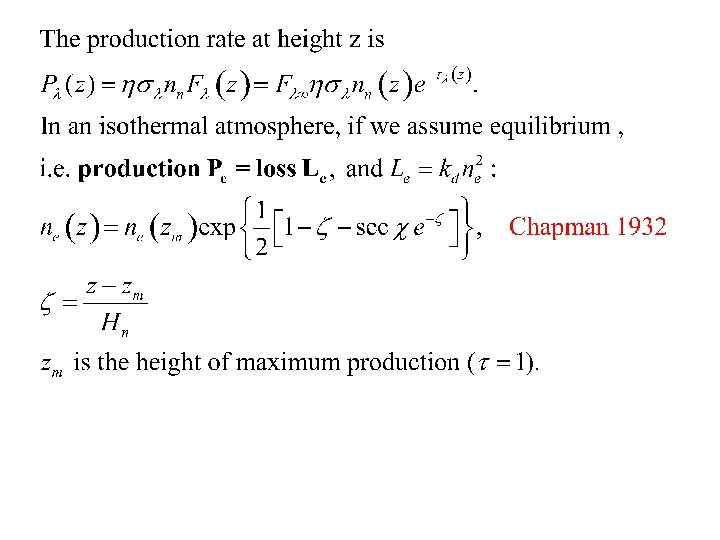Absorption of Solar Radiation vs. Height and Species